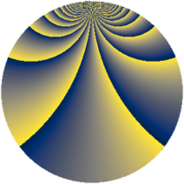# Properties

 Label 2240.2.biLevel $2240$ Weight $2$ Character orbit 2240.bi Rep. character $\chi_{2240}(1247,\cdot)$ Character field $\Q(\zeta_{4})$ Dimension $144$ Sturm bound $768$

# Related objects

## Defining parameters

 Level: $$N$$ $$=$$ $$2240 = 2^{6} \cdot 5 \cdot 7$$ Weight: $$k$$ $$=$$ $$2$$ Character orbit: $$[\chi]$$ $$=$$ 2240.bi (of order $$4$$ and degree $$2$$) Character conductor: $$\operatorname{cond}(\chi)$$ $$=$$ $$40$$ Character field: $$\Q(i)$$ Sturm bound: $$768$$

## Dimensions

The following table gives the dimensions of various subspaces of $$M_{2}(2240, [\chi])$$.

Total New Old
Modular forms 816 144 672
Cusp forms 720 144 576
Eisenstein series 96 0 96

## Trace form

 $$144q + O(q^{10})$$ $$144q - 48q^{17} + 48q^{25} + 48q^{65} + 48q^{73} - 144q^{81} + 48q^{97} + O(q^{100})$$

## Decomposition of $$S_{2}^{\mathrm{new}}(2240, [\chi])$$ into newform subspaces

The newforms in this space have not yet been added to the LMFDB.

## Decomposition of $$S_{2}^{\mathrm{old}}(2240, [\chi])$$ into lower level spaces

$$S_{2}^{\mathrm{old}}(2240, [\chi]) \cong$$ $$S_{2}^{\mathrm{new}}(40, [\chi])$$$$^{\oplus 8}$$$$\oplus$$$$S_{2}^{\mathrm{new}}(160, [\chi])$$$$^{\oplus 4}$$$$\oplus$$$$S_{2}^{\mathrm{new}}(280, [\chi])$$$$^{\oplus 4}$$$$\oplus$$$$S_{2}^{\mathrm{new}}(320, [\chi])$$$$^{\oplus 2}$$$$\oplus$$$$S_{2}^{\mathrm{new}}(1120, [\chi])$$$$^{\oplus 2}$$